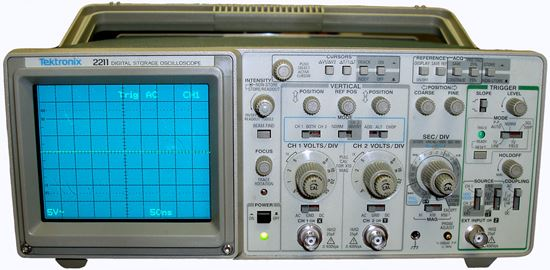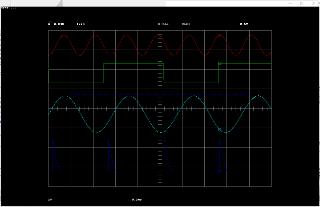## A "new" vintage Oscilloscope on my workbench :-)

For issues with communication ports, protocols, etc.
D.J.Peters
Posts: 7659
Joined: May 28, 2005 3:28

### A "new" vintage Oscilloscope on my workbench :-)

To day I got an vintage Tektronix 2211 50 MHz Digital Analog Oscilloscope from eBay (~20\$).Of course all this old (~1989) Tektronix and other Oscilloscopes does not have a USB interface
but many of them have a RS232C or centronics interface to plot the channels on pen plotters or printers.

I recorded with FreeBASIC the serial interface (9600 baud) from the device and the result are a HP-GL plotter file.

With a tiny HP-GL decoder in FreeBASIC you can plot this kind of data easly.

If you like to test it be sure the 4 channel demo file "PLOT.hpgl" are in the same folder.

Joshy

right click and save as: PLOT.hpgl

file: hpglplot.bas

Code: Select all

`'#define DEBUG#ifdef DEBUG #define DPRINT(msg) open err for output as #99:print #99,msg:close #99#else #define DPRINT(msg) :#endifconst HPGL_FILE = "PLOT.hpgl"function getText(aLine as string,byref iPos as integer) as string  var txt = ""  while aLine[iPos]<>asc(";") andalso aLine[iPos]<>asc(",")    txt &= chr(aLine[iPos])    iPos+=1  wend  iPos+=1  'DPRINT("txt: " & txt)  return txtend functionfunction getNumber(aLine as string,byref iPos as integer) as single  var number = getText(aLine,iPos)  return val(number)end function'' main'ChDir EXEPath()var FileName = HPGL_FILEvar hFile = FreeFile()if Open(FileName,for binary,access read,as #hFile) then  print "error: can't read " & FileName & "!"   beep : sleep : end 1end ifvar nBytes = LOF(hFile)DPRINT("file size: " & nBytes)var buffer = new ubyte[nBytes]get #hFile,,*Buffer,nBytesclose #hFiledim as single ratio,xSize,ySizedim as integer iRead,iPen,scrW,scrH,xMin,xMax,yMin,yMax,xPos,yPos,xOld,yOlddim as boolean scrActive,penActive,labActivescreeninfo scrW,scrHscrW*=0.75 : scrH*=0.75while iRead<nBytes  dim as string aLine '= ""  while right(aLine,1)<>chr(asc(";"))    if buffer[iRead]<>3 then      aLine &= chr(buffer[iRead])    end if      iRead+=1  wend    var aCmd = left(aLine,2)  var iPos=2  select case aCmd  case "DF" ' set default    penActive=false ' no active pen    labActive=false ' no active label    iPen = 1    xMin=0:xMax=scrW    yMin=0:yMax=scrH    xOld=0:yOld=0    xPos=0:yPos=0    DPRINT("reset to default")  case "SP" ' set pen #     iPen = getNumber(aLine,iPos)    DPRINT("set pen color " & iPen)  case "SC" ' scale    xMin = getNumber(aLine,iPos)    xMax = getNumber(aLine,iPos)    yMin = getNumber(aLine,iPos)    yMax = getNumber(aLine,iPos)    DPRINT("scale " & xMin & "," & xMax & "," & yMin & "," & yMax)     if scrActive=false then      screenres scrW,scrH      scrActive=true    end if      if scrActive then window (xMin,yMin)-(xMax,yMax)  case "PU" ' pen up    penActive=false    if aLine[iPos]=asc(";") then      DPRINT("pen up")     else      xOld=getNumber(aLine,iPos)      yOld=getNumber(aLine,iPos)      DPRINT("pen up at " & xPos & "," & yPos)     end if  case "PD" ' pen down    penActive=true    if aLine[iPos]=asc(";") then      DPRINT("pen down")      else      xPos=getNumber(aLine,iPos)      yPos=getNumber(aLine,iPos)      xOld=xPos:yOld=yPos      DPRINT("pen down at " & xPos & "," & yPos)       'pset (xPos,yPos),iPen    end if      case "PA" ' plot absolute    xOld=xPos:yOld=yPos    xPos=getNumber(aLine,iPos)    yPos=getNumber(aLine,iPos)    if penActive then      DPRINT("plot to " & xPos & "," & yPos)       if labActive then        line (xOld,yOld)-(xPos,yPos),iPen      else        line (xOld,yOld)-(xPos,yPos),7      end if      else      DPRINT("move to " & xPos & "," & yPos)     end if    case "SR" ' set relative character size    xSize=getNumber(aLine,iPos)    ySize=getNumber(aLine,iPos)    'DPRINT("char size " & xSize & "," & ySize)       case "LB" ' label    labActive=true    var sLabel = getText(aLine,iPos)    draw string (xOld,yOld),sLabel,15    DPRINT("label: " & sLabel)  case else    DPRINT("unsupported HP-GL command " & aCmd)    beep:sleep  end selectwendwindowtitle "done ..."deallocate buffersleep`

click to enlarge: